# Set Partition Problems

This is my first post of 2019 and my first post in a while. There was one posted a few months ago, but not really geared towards the algorithms and learning focus of the site. I have been doing a lot of coding in my spare time, but honestly life has just gotten in the way. Its not a bad thing, but life is life and sometimes I have to prioritize the things. In particular, I have been having ideas and actually coding things up but the time it takes to clean up code, write a blog entry and finding a nice way to visualize these things has been something that I haven’t been able to really focus on as much as I’ve wanted to.

That said, I want to talk to you about the Set Partition Problem today. You can go to Wikipedia to get more information about this problem, but I will give you a brief introduction to it and then talk about two different approaches to it. The problem assumes that we are given as input a (multi)-set S. The reason we say it is a multi-set and not a simple set is because we can have the same element appear multiple times in the set. So if S1 = {2} and S2 = {2, 2}, then although as sets they are both equal to the set {2} = S1, as multi-sets allow for multiple instances of an element. The elements of S are assumed to be positive integers.

So given this multi-set S, we ask the question of can the elements of S be divided into two smaller multi-sets, C1 and C2 where

• C1 [union] C2 = S
• C1 [intersect] C2 = [empty set]
• [Sigma]_[x in C1] = [Sigma]_[x in C2].C1 [union] C2 = S, C1 [intersect] C2 = [empty set], and [Sigma]_[x in C1] = [Sigma]_[x in C2]

The first two bullets above say that the sets C1 and C2 form a partition of S. The third bullet says that the sums of the elements in the two children multi-sets are equal.

This problem is known to be NP Complete. This means that it is one of the more difficult decision problems. Because of this finding an algorithm that solves this problem exactly will generally take a long running time. And finding an algorithm that runs quickly will more than likely be incorrect in some instances.

I will show you two approaches to this problem. One is based on Dynamic Programming (DP) and one is based on Greedy Algorithms. The DP version solves the problem exactly but has a slow running time. The greedy algorithm is fast but is not guaranteed to always give the correct answer.

Dynamic Programming is based on the principle of optimality. This says that in order to have a correct solution to the overall problem, we need optimal solutions to all subproblems of this problem. This is done by keeping track of a table which can be used for looking up these subproblems and the optimal solutions to these subproblems.

For this problem, we will build a table where the rows represent the final sums and columns represent subsets of the given multi-set (containing the first 0…n elements). The question we are repeatedly asking is “can we find a subset of the set in this column whose sum is exactly the given rowsum?” Here is an example of the DP algorithm on the multi-set {5, 6, 5, 6, 7}.

Greedy Algorithms are generally based on sorting the elements based on some principle and using that to try to answer the underlying question. The main problem with this approach is that it is very short sided because they do not look at the overall picture. Such algorithms are known for finding local optima that are not always globally optimal. The benefit to these problems though is that they are generally easier to code and easier to understand.

For the Set Partition Problem , the Greedy approach is to sort elements in descending order. Once this is done, the goal is to keep two subsets while iterating through the array, adding the element to the smaller of the two sets whenever possible.

For more examples, check out Set Partition Problems

# ID3 Algorithm Decision Trees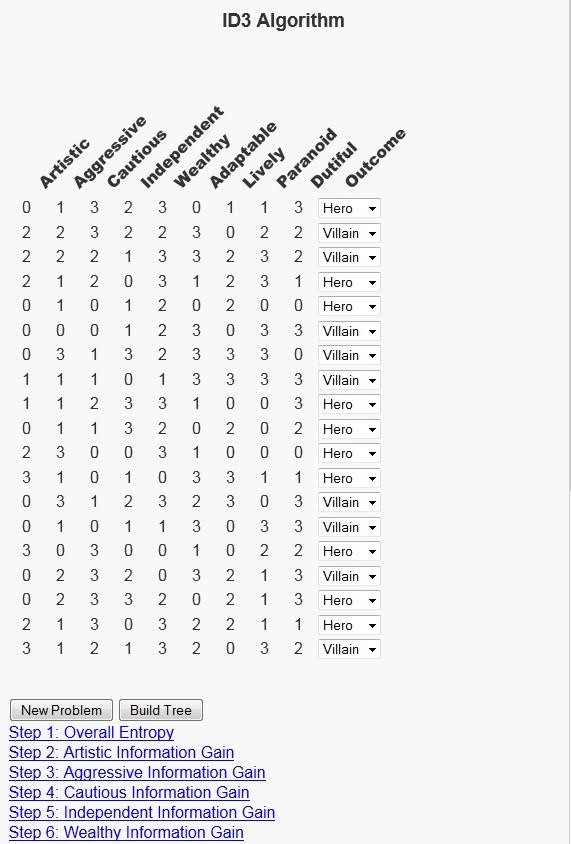As I grow LEARNINGlover.com, I’m always thinking of different ways to expose my own personality through the site. This is partially because it is easier for me to talk about subjects where I am already knowledgeable, but it is more-so being done to help make some of these algorithms and concepts I encode more understandable, and sometimes relating foreign concepts to everyday life makes them easier to understand.

Today, I’d like to write about decision trees, and the ID3 algorithm for generating decision trees in particular. This is a machine learning algorithm that builds a model from a training data set consisting of a feature vector and an outcome. Because our data set consists of an outcome element, this falls into the category of supervised machine learning.

The model that the ID3 algorithm builds is called a decision tree. It builds a tree based on the features, or columns of the data set with a possible decision corresponding to each value that the feature can have. The algorithm selects the next feature by asking “which feature tells me the most about our data set?” This question can be answered first by asking how much “information” is in the data set, and then comparing that result with the amount of information in each individual feature.

In order to execute this algorithm we need a way to measure both the amount the information in outcomes of the overall data set as well as how much each feature tells us about the data set. For the first, we will use entropy, which comes from the field of information theory and encoding. Entropy is based on the question of how many bits are necessary to encode the information in a set. The more information, the higher the entropy, and the more bits required to encode that information. Although we are not encoding, the correlation between high information and high entropy suits our purposes.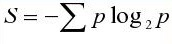To understand how much each feature tells us about the outcomes of the data set we will build on the concept of entropy to define the information gain of a feature. Each feature has multiple options, so the dataset can be partitioned based on each possible value of this feature. Once we have this partition, we can calculate the entropy of each subset of the rows of data. We define the information gain of a feature as the sum over all possible outcomes of that feature can have of the entropy of that outcome multiplied by the probability of that outcome.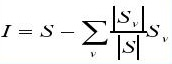To illustrate this algorithm, I decided to relate it to the question of whether we think of a character in a novel as a hero or villain. This is interesting because I try to read at least one book a month and as I’m reading, I often find myself asking this question about characters based on the traits of the characters as well as characters I’ve read about. In order to build an interactive script for this problem, I considered 25 possible character traits that could be present. A subset of these 25 character traits will be selected and a row will be generated grading a fictional character on a scale of 0 to 3 (0 meaning that they do not possess the trait at all, 3 meaning that the trait is very strong in their personality), and users will be asked whether they think a character with the given character traits should be listed as a hero or a villain. Then there is a button at the bottom of the script with the text “Build Tree” that executes the ID3 Algorithm and shows a decision tree that could be used to reach the set of decisions given by the user.

The possible features are:
Abstract, Adaptable, Aggressive, Ambition, Anxiety, Artistic, Cautious, Decisive, Honesty, Dutiful, Fitness, Intellect, Independent, Introverted, Lively, Open-minded, Orderly, Paranoid, Perfectionist, Romantic, Sensitive, Stable, Tension, Warmth and Wealthy

Once users select the option to build the tree, there will be several links outlining each step in the process to build this tree. These links will allow for users to expand the information relating to that step and minimize that information when done. Hopefully this will help users to understand each step more. I must say that as much fun as it has been writing this program, there were several questions when trying to explain it to others. Hopefully users get as much fun from using this tool as I had in creating it. As always feel free to contact me with any comments and or questions.

Ok, so here’s a link to the ID3 Algorithm Page. Please check it out and let me know what you think.

# The A* Algorithm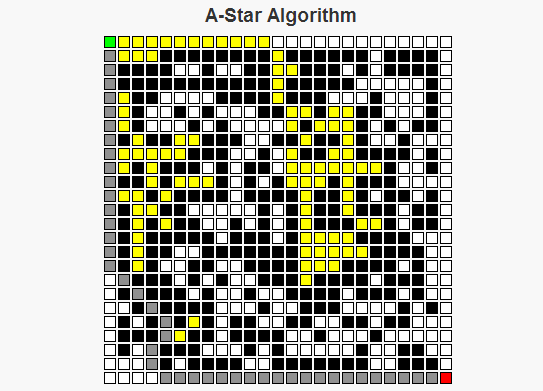As a child I remember traveling on road trips, sitting in the back of a car trying to do my best to keep myself busy for what would occupy the next six to ten hours of my life. One of the things I grew to like were the simple maze books that were sold on the magazine racks at some of the gas stations where we’d stop for food. There are two basic strategies I employed for solving these mazes: For simpler mazes, I could generally just take a look at the overall maze structure, decide upon a path through the maze, then write a path without any mistakes. For more complex mazes though, I would generally begin a route that looks the most promising. If that route reaches a point where I can see that it will be impossible to finish, then I’d go back to where the decision was made, exclude the route I had just tried, and select the “most promising” remaining route. I’d continue this process until I had completed the maze, or until it became simple enough for me to solve the maze using only my memory.

The A* Algorithm works in a similar manner to the second approach I just described. We begin at a starting point, and consider where to move next from that starting point. The set of possible options for this next move is determined by the neighbor function for a given cell. For each neighbor the algorithm estimates the length of the route through that cell by calculating the sum of two values, f(n) = g(n) + h(n), where

g(n) is the (known) cost to travel from the starting point to the cell n.
h(n) is the (approximate) cost to travel from the cell n to the final cell.

The sum g(n) + h(n) allows us to approximate the total cost of a route through the cell n.

The A* algorithm begins at the starting position of the maze. There are two sets we will be considering throughout the process of determining the optimal route, called the closedSet and the openSet. The elements of closedSet are the nodes whose total distance from the starting position has been calculated, whereas the elements of openSet represents nodes whose total distance is still under consideration. There is also a map called prev which is used to reconstruct the path. Below is how the algorithm operates:

A* Algorithm Pseudocode
closedSet is the empty set
openSet = {start}
prev is the empty set

While there are still elements in openSet,
Find the element c* in openSet with the lowest value f(c).
If c* is the target position
Reconstruct the path.
Else If c* is not the target position,
Remove c* from openSet
For each neighbor n of c* that is not in closedSet,
Calculate a temporary g value, temp_g(n) = g(c*) + dist(c*, n).
If n is not in openSet, or if n is in openSet and temp_g(n) < g(n),                     Set prev(n) = c*                     Set g(n) = temp_g(n)                     Set f(n) = g(n) + h(n).                     if n is not in openSet                          add n to openSet.      End If End While End AlgorithmAn important question becomes what makes a good heuristic function to approximate the distance to the goal. This can lead to an in depth discussion based on the word "good", but the necessary condition for any heuristic is that it NEVER over-estimates the cost of the path from the cell to the goal. Some examples of possible heuristics for mazes are the Euclidean Distance (the square root of the sum of the squares of the horizontal and vertical differences in distances, i.e. dE(x, y) =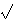(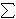i(xi – yi)2) and TaxiCab Distance (the sum of the differences in the horizontal and vertical dimensions, i.e. dT(x, y) =i|xi – yi|). Both of these are feasible metrics for heuristics on a maze. Other herusitics, like h(n) = 0 for all n are possible, but doing this would make the algorithm treat all cells equally and ignore the heuristic part of the A* algorithm, turning it into Dijkstra’s algorithm.

I’ve written a script that generates random mazes and uses the A* algorithm to find the optimal path through this maze. For this script, I used the taxicab distance heuristic.

Check it out and let me know what you think.

# Learn About “the Other” AlgebraWhen I visit family for the holidays, the topic of my being a mathematician always seems to come up, and there’s always a child in the family struggling with maths, and when I ask the subject of their struggles the word “algebra” is always the culprit. I’ll save for another post my ideas on how this subject should be taught in high school and some of the main problems facing students.

I want to concentrate this post on a topic that few outside the mathematical world know about, but which many inside this world (myself included) hold dearly – the topic of modern or abstract algebra. I refer to this as “the other” algebra because a general conversation about the word “algebra” will generally revolve around concepts such as systems of equations, slopes, intercepts, intersection, rise-over-run, point-slope, and other terminology that limits algebra to a specific domain (the set of real or complex numbers) while at the same time ignoring the underlying beauty associated with this area.

I wrote previously about the area of set theory and the beauty associated with taking math out of the scope of a basic number line and into a much more undefined space. Abstract algebra is a continuation of set theory where in addition to our set, we have a (binary) operation defined on any two elements of this set. The inclusion of this binary operation allows us to consider several different structures based on the properties that this binary operation holds.

The structures I’d like to write about today are called groups. A group is a set along with an operation (or function) defined on any two elements of the set with the following properties:
– It is closed. This means that any time we run this function on two elements on the set, the function gives us a member of the set. In mathematical terms, for all a, b in the set A, f(a, b) must also be a member of A.
– There is an identity element. An identity element is defined as an element where is we include it in the binary operator with any other element, the operator will always return the other element. So if the element i is the identity element, then f(i, a) = a and f(a, i) = a for any other a in the set A. Any group must have an identity element.
– Every element has an inverse. Inverse elements are based on the identity element. What the inverse says is that for every element, there is a way to use the binary operator to get to the identity element. So for all elements a in the set A, there is an element b in the set A such that f(a, b) = i, where i is the identity element.
– The binary operator is associative. I described the associative property when I discussed the functions and relations of set theory. A function is associative if the way we group things (aka associate them) doesn’t matter. This means that for any elements a, b, and c of the set A, f(f(a, b), c) must be the same as f(a, f(b, c)).

If these four properties hold for a set A and a binary function f, then we say that the pair (A, f) is a group. We will generally use a common notation such as a · b, or a * b or simply ab to represent f(a, b).

Another important concept in group theory is the idea of a Cayley table. These are similar to multiplication tables that we drew out when we were first learning our “times tables”. For a group with n elements, we form a table with n rows and n columns. Each element of the group is written out to the left of each row and above each column (so really we can think of it as an n+1 by n+1 table with the first row and column being descriptive rows). Each cell of the table is the binary operator applied to the two elements indicated by the row and column (with an understanding of whether we have row before column or vice versa). Obviously, we can only do this for finite groups as we cannot write out all the elements of an infinite set.

The script I’ve added is a tester to allow users to input the information for a possible group (size, name of each element, and a Cayley table) and with this information the user is informed whether or not it forms a group. If it does not form a group, the reasons why it does not form one are also given. There are also some sample groups given to give insight into this area.

# Understanding Bayes’ Theorem

I’ve finished a script that helps understand Bayes’ Theorem.

If we have a set of mutually exclusive (aka non-overlapping) sets Bi for i{0, 1, 2, …, n} for some integer n, then the union of these sets forms a sample space. Lets call the sample space S. Suppose that we also have some set (also known as an event) A which is also a subset of S. Bayes’ Theorem considers the probability that one of these mutually exclusive events (one of the Bi‘s) caused the observed event (A).

This probability can be calculated by the formula

 Pr(Bj | A) = Pr(Bj) Pr(A | Bj)Pr(Bi) Pr(A | Bi)

The theorem helps us determine the the probability of the event Bj given A, or in more plain English, the probability that the event Bj is the cause that gives rise to the observed event A. The numerator is given by the product of of the probability of the causal event (Pr(Bj) times the conditional probability of the observed event given the causal event (Pr(A | Bj)). This numerator could be replaced by its equivalent statement of the set A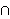Bj. Likewise, the denominator the sum (over all the causal events) of the probaility of each causal event times the conditional probability of the observed event given that particular causal event. Each term in this denominator could be replaced b its equivalent staetment ABi, which when summed give the total probability of A because each pair of the Bj‘s is mutually exclusive. So we are able to replace the probability of A withPr(Bi) Pr(A | Bi) because of the fundamental law of probability.

An example that would use Bayes’ Theorem is analyzing the results of an election. The set of mutually exclusive events could be membership in a political party (Democrat, Republican, or Independent). The observed event could be the election of an individual. And the conditional distributions could be the percentage of each party that voted for this individual. If we want to calculate how significant each party was to the individual’s election, we’d use Bayes’ Theorem.

The script I’ve written to help understand Bayes’ Theorem works as follows:
– A set of mutually exclusive sets is randomly generated (the number of sets also varies). These sets are called Bi for i(0, …, n}.
– A set A is randomly generated from the union of the Bi‘s.
– A table is displayed showing:
Pr(Bi) for each i on line 1.
Pr(A | Bi) for each i on line 2.

– The user is given the option to select which of the mutually exclusive sets they would like to use to calculate the probability that this set caused the event A.
– Once a set is chosen, the user clicks the “Calculate Conditional” button and Bayes’ Theorem gives the result.
– If the “show work” checkbox was checked, then the steps used in this calculation are also shown.
– All work is done using fractions to give an idea of where the numbers come from.

Other Blogs that have covered this topic:
Better Explained
Bayes’ Theorem-qed